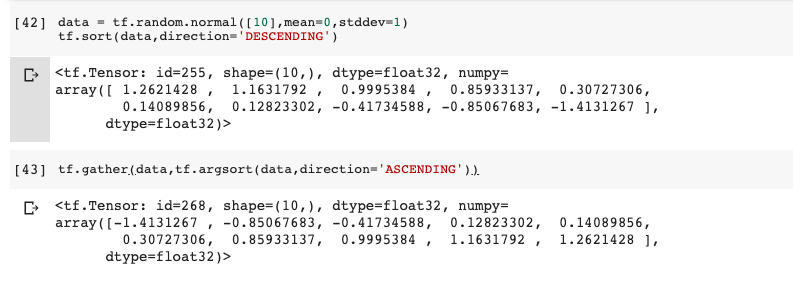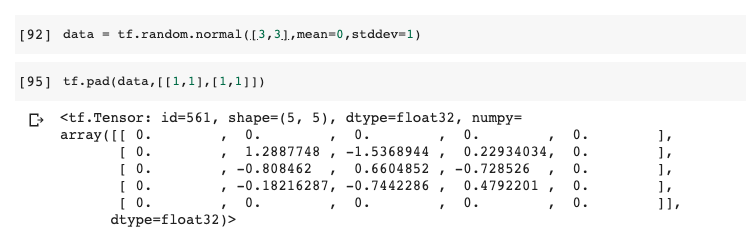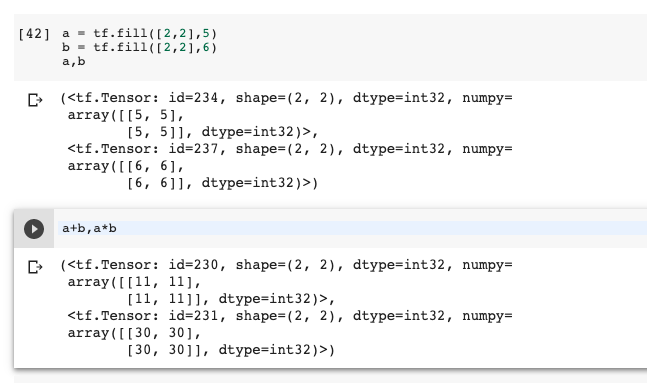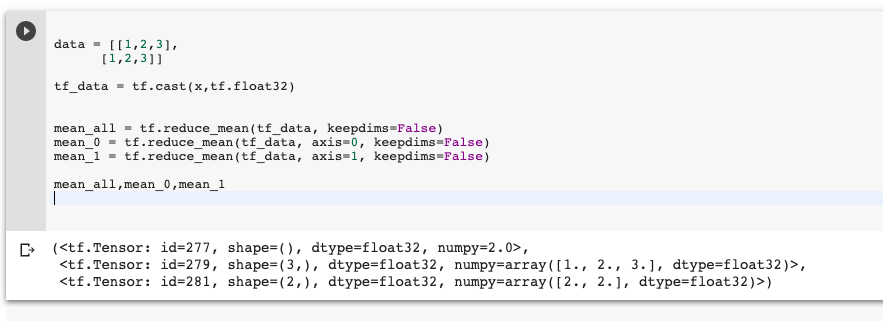DAY 4
0
Google Developers Machine Learning

[Day-4] Tensorflow 基本語法 - Part III

Sort

#Example for sort
data = tf.random.normal(,mean=0,stddev=1)
tf.sort(data,direction='DESCENDING')
#or
tf.gather(data,tf.argsort(data,direction='ASCENDING'))#Example top_k
top_data = tf.top_k(data,5)
#Get indies or values
top_data.indies
#or
top_data.valuesdata = tf.random.normal([3,3],mean=0,stddev=1)Clipping

#Example clipping
tf.clip_by_value(data,0,1)
#range if number less or above fix at 0 or 1

基本數學運算

• Element wise: 直接對相對應的元素相加，Ex: +-
• Matrix wise: 矩陣相乘，Ex: [6,32,4] @ [6,4,6] = [6,32,6]
• Dimention wise: 針對特定dimention做運算。Ex: 對整個dimention做reduce_mean。
#Example for element wise
a = tf.fill([2,2],5)
b = tf.fill([2,2],6)
a+b,a*b#Example for Matrix wise
a = tf.fill([2,32,4],5)
b = tf.fill([2,4,6],6)
(a@b).shape # or tf.matmul(a,b)
#Output:TensorShape([2, 32, 6])

#Example for dimension wise
data = [[1,2,3],
[1,2,3]]

tf_data = tf.cast(x,tf.float32)

mean_all = tf.reduce_mean(tf_data, keepdims=False)
mean_0 = tf.reduce_mean(tf_data, axis=0, keepdims=False)
mean_1 = tf.reduce_mean(tf_data, axis=1, keepdims=False)

mean_all,mean_0,mean_1